# Python Tutorials: Plotting the Pixels on a Matplotlib in Python

Written by | 0 Comments | 4870 Views

Python Tutorial: In this article, we will Cover Plotting the Pixels on Matplotlib GUI in Python. Matplotlib is more than just plotting the graphs. Matplotlib is more than just plotting the graphs.

In the previous section, we have seen how to plot the graphs in Matplotlib. However, Matplotlib is more than just plotting the graphs. We can also plot the pixels on a Matplotlib GUI. There is a particular function that can show the image on Matplotlib main window. This function is called the imshow() function.

However, the question arises of how this happens. For showing an image on Matplotlib, we have to convert the image into NumPy. NumPy is a special module in python used for scientific calculation and for calculating the pixel values. We will see in-depth about the NumPy module. Here we will convert the image into NumPy, and except for giving the data in the list data structure, we will provide it in NumPy array data structures.

Following is the method to show the image:

 import matplotlib.pyplot as plt import matplotlib.image as img import NumPy as np image = img.imread('/home/lalatoofani/Desktop/pylogo.png') print(image)

If we print the image, it will be printed as a NumPy array; the following is the output of the above-given code:

 Output   [[[1. 1. 1. 1.]   [1. 1. 1. 1.]   [1. 1. 1. 1.]   ...   [1. 1. 1. 1.]   [1. 1. 1. 1.]   [1. 1. 1. 1.]]    [[1. 1. 1. 1.]   [1. 1. 1. 1.]   [1. 1. 1. 1.]   ...   [1. 1. 1. 1.]   [1. 1. 1. 1.]   [1. 1. 1. 1.]]    [[1. 1. 1. 1.]   [1. 1. 1. 1.]   [1. 1. 1. 1.]   ...   [1. 1. 1. 1.]   [1. 1. 1. 1.]   [1. 1. 1. 1.]]    ...    [[1. 1. 1. 1.]   [1. 1. 1. 1.]   [1. 1. 1. 1.]   ...   [1. 1. 1. 1.]   [1. 1. 1. 1.]   [1. 1. 1. 1.]]    [[1. 1. 1. 1.]   [1. 1. 1. 1.]   [1. 1. 1. 1.]   ...   [1. 1. 1. 1.]   [1. 1. 1. 1.]   [1. 1. 1. 1.]]    [[1. 1. 1. 1.]   [1. 1. 1. 1.]   [1. 1. 1. 1.]   ...   [1. 1. 1. 1.]   [1. 1. 1. 1.]   [1. 1. 1. 1.]]]

It is a nested array. Each nested array shows one pixel as an RGBA value. This NumPy array, when subjected to Matplotlib, will be plotted on a window:

 import matplotlib.pyplot as plt import matplotlib.image as img import NumPy as np image = img.imread('/home/lalatoofani/Desktop/pylogo.png') plt.imshow(image) plt.show()

In the first line, we import Matplotlib to plot the graph, and then we import the image module of Matplotlib to read the image file from the local device.

The imshow() function plot the pixel on the main window, and last, we show the image.

The above code output the following image.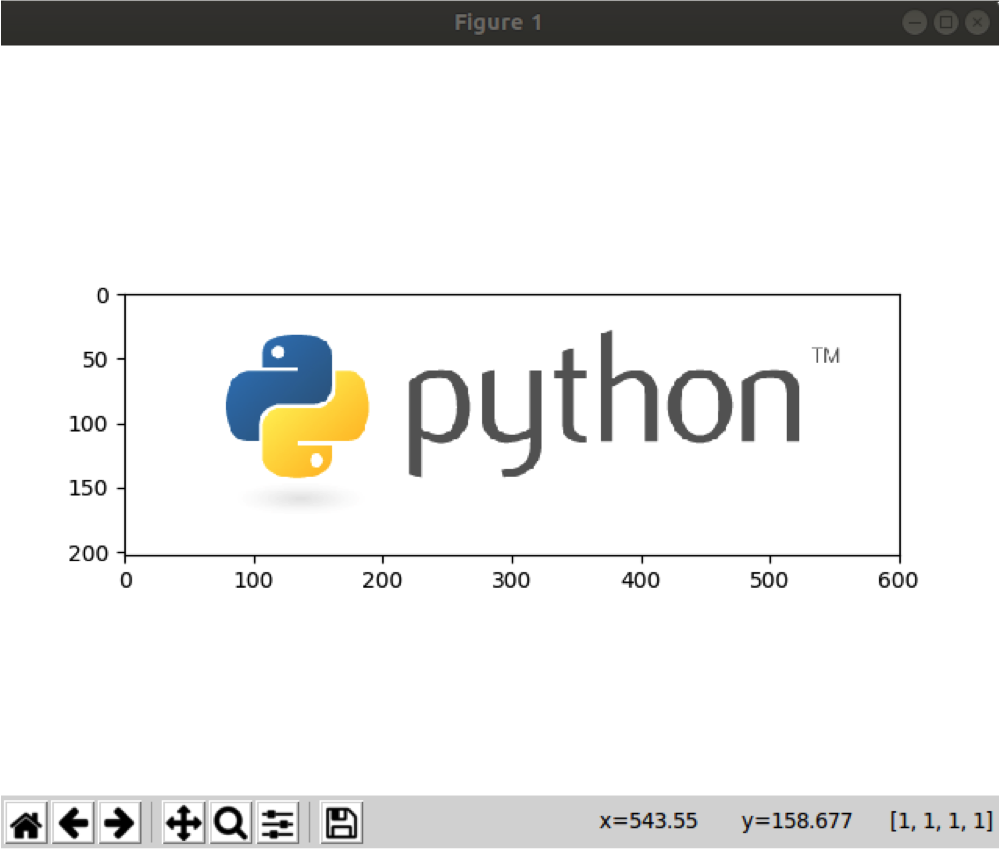Figure 1

In Image processing and object detection, we identify the image with its histogram. Just like the height and attributes of different persons are different similarly, the object has different histograms. Histograms are the pixel height of an object. Now let's suppose we have an image of a hand as follows we will first plot the image and then its histogram.

Following is the code of outputting the histogram:

 import matplotlib.pyplot as plt import matplotlib.image as img import NumPy as np image = img.imread('/home/lalatoofani/Desktop/hand.jpg') plt.imshow(image) plt.show()

The output will be the following: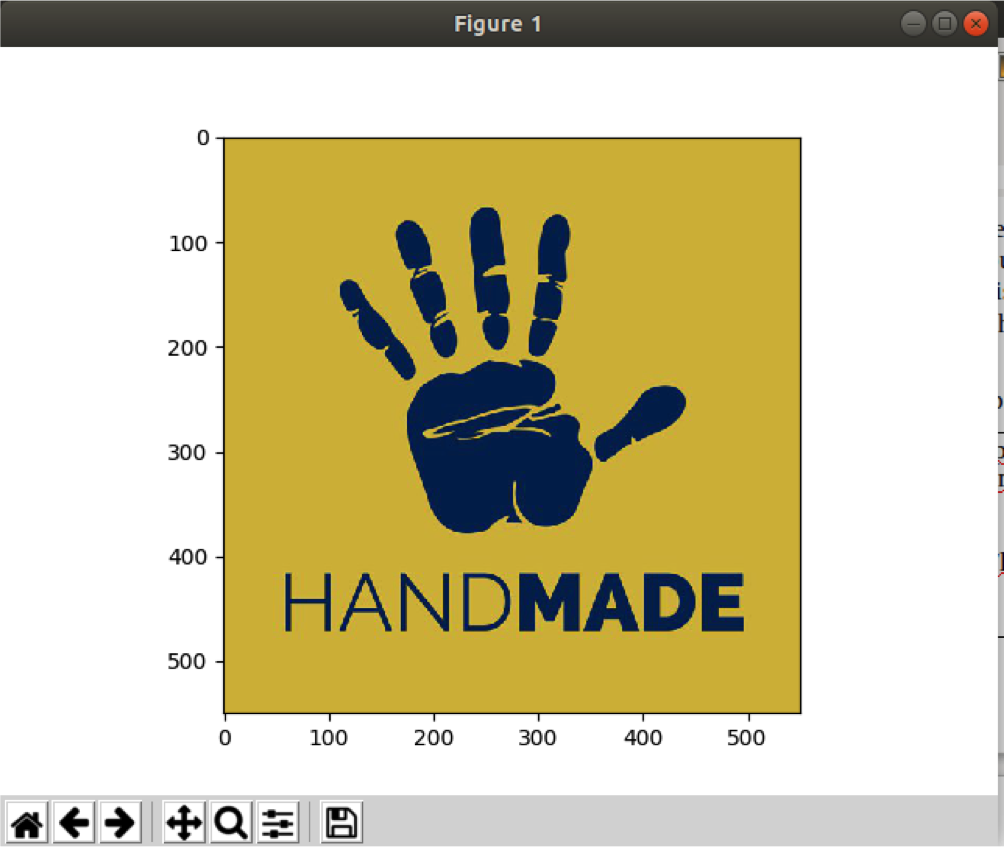Figure 2

Now to make a histogram of the above image following code is to be followed:

 import matplotlib.pyplot as plt import matplotlib.image as img import NumPy as np image = img.imread('/home/lalatoofani/Desktop/hand.jpg') plt.hist(image.ravel(), bins=256, range=(0.0, 1.0), fc='k', ec='k') plt.show()

The output of the above code is as follows: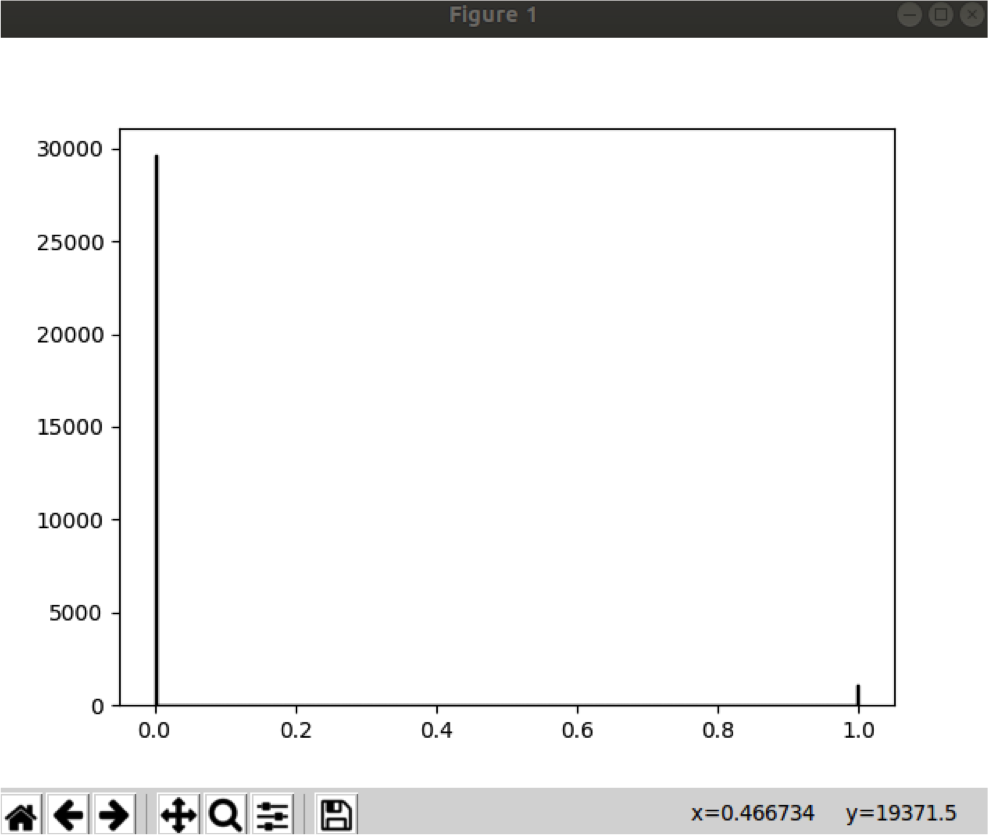Figure 3

As we can see, the image is not too dense. That is why the histogram is clear. If the image is denser, the graph of a histogram is also dense. We will see in much detail about the histogram when we will learn about OpenCV.

Plotting with Animation:

As I have already stated, plotting in Matplotlib is not just plotting a graph but also way more than that. We can plot a graph, plot images, medical images, can make 3d plots, and now we will animate the simple line graph, which can make it more effective.

Following is the code of animating the graph:

 import NumPy as np import matplotlib.pyplot as plt from matplotlib.animation import FuncAnimation fig, ax = plt.subplots() x, y = [], [] g_plot,= plt.plot([], [], 'g-', animated=True) def init_func():     ax.set_xlim(0, 2*np.pi)     ax.set_ylim(-1, 1)     return g_plot, def update_func(frame):     x.append(frame)     y.append(np.sin(frame))     g_plot.set_data(x, y)     return g_plot, animation_start = FuncAnimation(fig, update_func, frames=np.linspace(0, 2*np.pi, 128),                     init_func=init_func, blit=True) plt.show()

First, we will see what is happening in the code. First, as usual, we imported the pyplot library from Matplot; we also imported the NumPy module because we will plot the sin wave on a window. In the third line, we imported the function method from the Matplot library.

We initialize the subplot and also initialize the x and y as an empty list data structure. Then we define an init function, which assigns the x limit of the gram and y limit. Here the "y" limit is in the range of -1 and 1. Then there is another function, which is an update function that takes the frame. It will be updated on each successive cycle and plot the x and y on the Matplot lib window.

We append the data in x and y and set the data as x and y, and return the plot instance named as g_plot. Then we start the animation by calling the module func animation in which we have provided the figure instance, update_func, and its parameters. After this, we plot it in the graph. The graph will be updated every seconf and will be completed slowly, making an animated effect.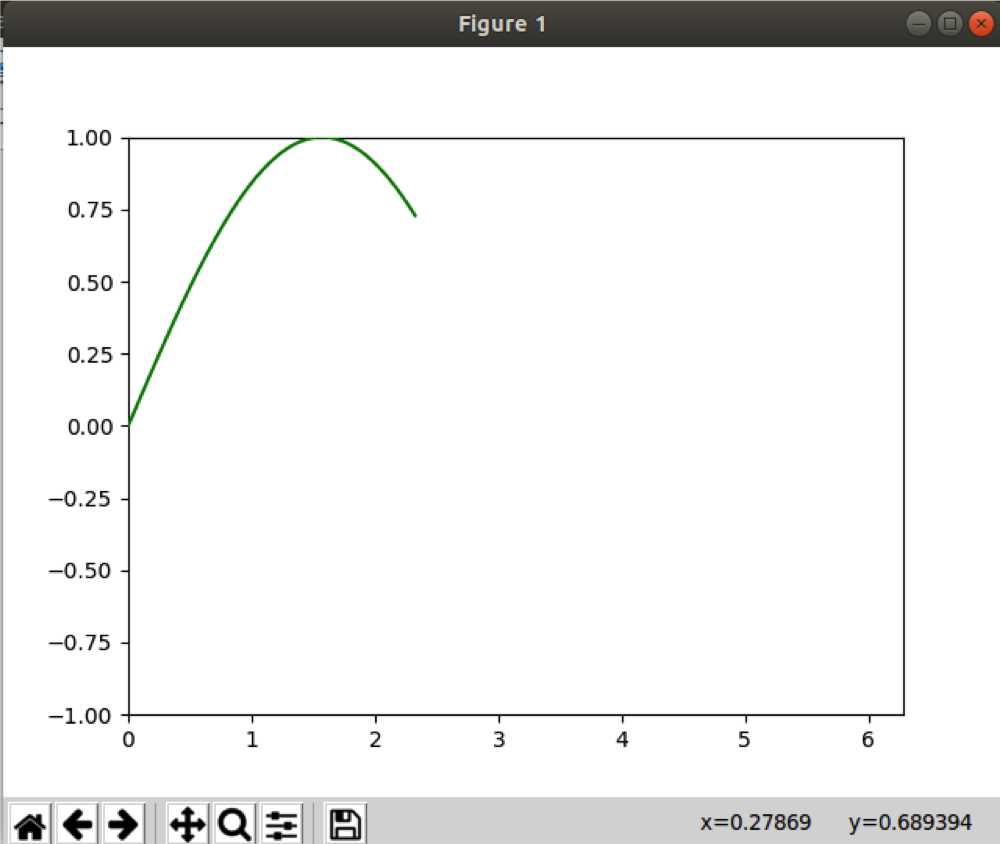Figure 4

The above image is taken just a few seconds after it started. In the end, the graph looks like this: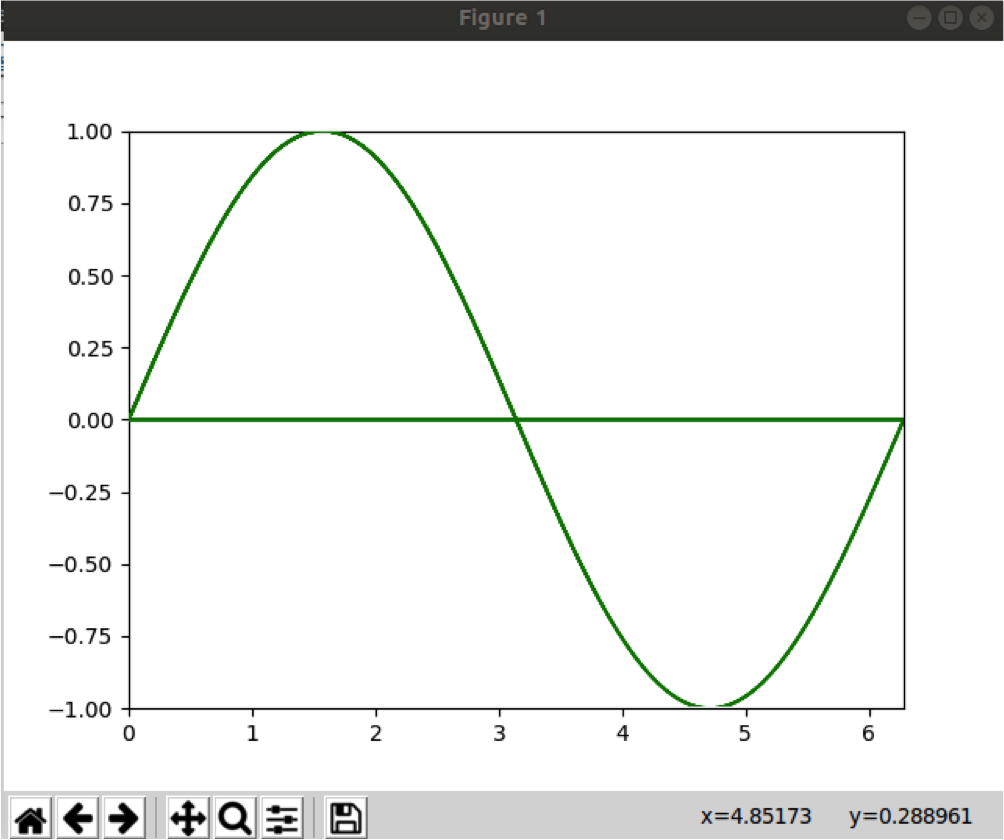Figure 5

This graph is made by using the scientific formula of creating the sine wave.

The Matplotlib is so vast that we can't completely cover it in just three articles. We can do a lot more. However, this is it for now, as I can't want to make it more complicated. Data visualization is the key to data science. Without data visualization, data science is nothing. So, make yourself efficient before we dive into data science.